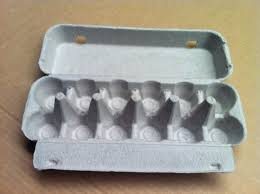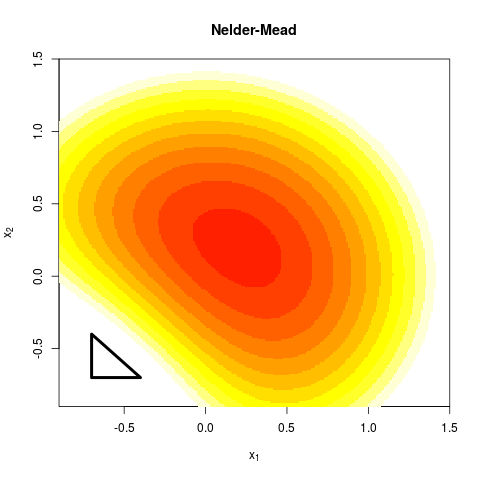School of Economics and Management
Beihang University
http://yanfei.site

## Discontinuous Functions

• The Newton Method requires first and second derivatives.
• If derivatives are not available the they can be approximated by Quasi-Newton methods.
• What if the derivatives do not exist?
• This may occur if there are discontinuities in the function.

• Suppose the aim is to optimize income of the business by selecting the number of workers.
• If too many workers are employed, they may be less efficient and the income of the company goes down.• Now suppose that there is a tax that the company must pay.
• Companies with less than 50 workers do not pay the tax.
• Companies with more than 50 workers do pay the tax.
• How does this change the problem?• The Nelder Mead algorithm is robust even when the functions are discontinuous.
• The idea is based on evaluating the function at the vertices of an n-dimensional simplex where n is the number of input variables into the function.
• For two dimensional problems the n-dimensional simplex is simply a triangle, and each corner is one vertex
• In general there are n + 1 vertices.

## A 2-dimensional simplex## Step 1: Evaluate Function

• For each vertex $${\mathbf x_j}$$ evaluate the function $$f({\mathbf x_j})$$
• Order the vertices so that $f({\mathbf x_1})\leq f({\mathbf x_2})\leq\ldots\leq f({\mathbf x_{n+1}}).$
• Suppose that the aim is to minimize the function, then $$f({\mathbf x_{n+1}})$$ is the worst point.
• The aim is to replace $$f({\mathbf x_{n+1}})$$ with a better point.

## A 2-dimensional simplex## Step 2: Find Centroid

• After eliminating the worst point $${\mathbf x_{n+1}}$$, compute the centroid of the remaining $$n$$ points ${\mathbf x_0}=\frac{1}{n}\sum_{j=1}^{n} {\mathbf x_j}.$
• For the 2-dimensional example the centroid will be in the middle of a line.

## Find Centroid## Step 3: Find reflected point

• Reflect the worst point around the centroid to get the reflected point.
• The formula is: ${\mathbf x_r}={\mathbf x_0}+\alpha({\mathbf x_0}-{\mathbf x_{n+1}}).$
• A common choice is $$\alpha=1$$.
• In this case the reflected point is the same distance from the centroid as the worst point.

## Find Reflected Point## Find Reflected Point## Three cases

1. $$f({\mathbf x_1})\leq f({\mathbf x_r})<f({\mathbf x_n})$$
• $${\mathbf x_r}$$ is neither best nor worst point
2. $$f({\mathbf x_r})<f({\mathbf x_1})$$
• $${\mathbf x_r}$$ is the best point
3. $$f({\mathbf x_r})\geq f({\mathbf x_n})$$
• $${\mathbf x_r}$$ is the worst point

## Case 1

In Case 1 a new simplex is formed with $${\mathbf x_{n+1}}$$ replaced by the reflected point $${\mathbf x_{r}}$$. Then go back to step 1.## Case 1## Case 2

In Case 2, $${\mathbf x_r}<{\mathbf x_1}$$. A good direction has been found so we expand along that direction ${\mathbf x_e}={\mathbf x_0}+\gamma({\mathbf x_r}-{\mathbf x_0}).$

A common choice is $$\gamma=2$$

## Case 2## Case 2## Choosing the Expansion Point

• Evaluate $$f({\mathbf x_e})$$.
• If $$f({\mathbf x_e}) < f({\mathbf x_r})$$:
• The expansion point is better than the reflection point. Form a new simplex with the expansion point
• If $$f({\mathbf x_r})\leq f({\mathbf x_e})$$:
• The expansion point is not better than the reflection point. Form a new simplex with the reflection point.

## Keep Expansion Point## Keep Relection Point## Case 3

Case 3 implies that there may be a valley between $${\mathbf x_{n+1}}$$ and $${\mathbf x_{r}}$$ so find the contracted point. A new simplex is formed with the contraction point if it is better than $${\mathbf x_{n+1}}$$ ${\mathbf x_c}={\mathbf x_0}+\rho({\mathbf x_{n+1}}-{\mathbf x_0})$

A common choice is $$\rho=0.5$$

## Case 3## Valley## Find Contraction point## New Simplex## Shrink

If $$f({\mathbf x_{n+1}})\leq f({\mathbf x_{c}})$$ then contracting away from the worst point does not lead to a better point. In this case the function is too irregular a smaller simplex should be used. Shrink the simplex ${\mathbf x_i}={\mathbf x_1}+\sigma({\mathbf x_i}-{\mathbf x_1})$

A popular choice is $$\sigma=0.5$$.

## Egg Carton## Contraction Point is worst## New Simplex## Summary

• Order points
• Find centroid
• Find reflected point
• Three cases:
1. Case 1 ($$f({\mathbf x_1})\leq f({\mathbf x_r})<f({\mathbf x_n})$$): Keep $${\mathbf x_r}$$
2. Case 2 ($$f({\mathbf x_r}) < f({\mathbf x_1})$$): Find $$\mathbf x_e$$.
• If $$f({\mathbf x_e})<f({\mathbf x_r})$$ then keep $$\mathbf x_e$$
• Otherwise keep $$\mathbf x_r$$
3. Case 3 ($$f({\mathbf x_r})\geq f({\mathbf x_n})$$): Find $$\mathbf x_c$$
• If $$f({\mathbf x_c})<f({\mathbf x_{n+1}})$$ then keep $$\mathbf x_c$$
• Otherwise shrink• Find the minimum of the function $$f({\mathbf x})=x_1^2+x_2^2$$
• Use a triangle with vertices $$(1,1)$$, $$(1,2)$$, $$(2,2)$$ as the starting simplex